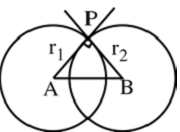# Orthogonal Circles

Two circles are said to be orthogonal if the tangent at their point of intersection are at right angles.Let the two circles be

x2 + y2 + 2g1x + 2f1y + c1 = 0

x2 + y2 + 2g2x + 2f2y + c2 = 0

The required condition for orthogonality is

2g1g2 + 2f1f2 = c1 + c2